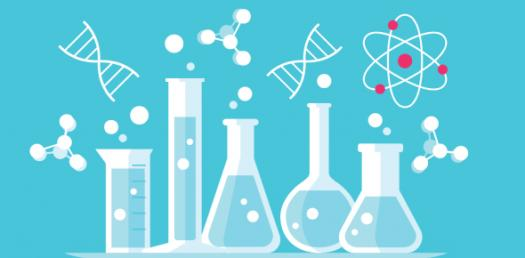# IBSL Chemistry Topic 3 Periodicity Multiple Choice Questions

13 Questions | Attempts: 1777
ShareSettings• 1.
For which element are the group number and the period number the same?
• A.

Li

• B.

Be

• C.

B

• D.

Mg

• 2.
Which properties of Period 3 elements increase from sodium to argon: I. nuclear charge II. atomic radius III. electronegativity
• A.

I and II only

• B.

I and III only

• C.

II and III only

• D.

I, II and III

• 3.
Which pair of elements reacts most readily?
• A.

Li + Br2

• B.

Li + Cl2

• C.

K + Br2

• D.

K + Cl2

• 4.
Which of the reactions below occur as written?
• A.

I only

• B.

II only

• C.

Both I and II

• D.

Neither I and II

• 5.
In the periodic table, the elements are arranged in order of increasing
• A.

Atomic number

• B.

Atomic mass

• C.

Number of valence electrons

• D.

Electronegativity

• 6.
Which one of the following series is arranged in order of increasing value?
• A.

The first ionization energies of: oxygen, fluorine, neon

• B.

The radii of: H- ion, H atom, H+ ion

• C.

The electronegativities of: chlorine, bromine, iodine

• D.

The boiling points of: iodine, bromine, chlorine

• 7.
Which property increases with increasing atomic number for both the alkali and the halogens?
• A.

Ionisation energies

• B.

Melting points

• C.

Electronegativities

• D.

• 8.
Which set of reactants below is expected to produce the most vigorous reaction?
• A.

Na(s) + Cl2(g)

• B.

K(s) + Cl2(g)

• C.

Na(s) + Br2(g)

• D.

K(s) + Br2(g)

• 9.
Which one of the following statements about the halogen group is correct?
• A.

First ionization energies increase from F to I.

• B.

Fluorine has the smallest tendency to be reduced

• C.

Cl2 will oxidize I- (aq)

• D.

I2 is a stronger oxidizing agent than F2

• 10.
Strontium is an element in Group 2 of the Periodic Table with atominc number 38. Which of the following statements about strontium is NOT correct?
• A.

Its first ionization energy is lower than that of calcium

• B.

It has two electrons in its outermost energy level

• C.

Its atomic radius smaller than magnesium

• D.

It forms a chloride with the formula SrCl2

• 11.
Which one of the following elements has the lowest first ionization energy?
• A.

Li

• B.

Mg

• C.

Na

• D.

Al

• 12.
Which element is most similar chemically to the element with 14 electrons?
• A.

Al

• B.

Ge

• C.

As

• D.

P

• 13.
0.01 mole samples of the following oxides were added to separate 1 dm^3 portions of water. Which will produce the most acidic solution?
• A.

Al2O3(s)

• B.

Na2O(s)

• C.

SiO2(s)

• D.

SO3(g)

## Related TopicsBack to top
×

Wait!
Here's an interesting quiz for you.